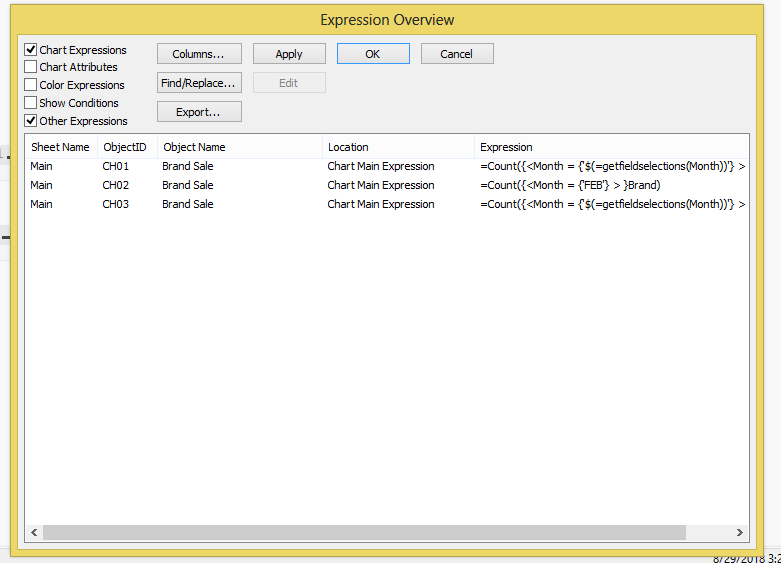New to QlikView

Discussion board where members can get started with QlikView.

New Contributor II

How to reference & use one chart expression in another expression of same chart?

Hi:

Can I ask as what's the right way to use one chart expression in defining another chart expression. What are the best practices around it and pitfalls?

Thanks,
Exan

Tags (1)
1 Solution

Accepted Solutions
Contributor

Re: How to reference & use one chart expression in another expression of same chart?

Hi,

One way to use expression in another chart expression is to create a variable,

For Ex if you have calculated Total Sales and you want that same expression in another chart so instead of using same expression in another chart use the variable name.

Pitfall is that if you have created a variable For ex if yo have created a variable for Total Sales and if you have made changes in variable and if yo use that variable in second chart so it will affect the both charts as the changes has been done in the variable.

PFA

Regards

Ritesh

6 Replies
Honored Contributor II

Re: How to reference & use one chart expression in another expression of same chart?

1. Copy and Paste

2. Keep all the expressions in a text file and load into target QVW.

I think these two are the ways. I prefer second point.

All the best.

Contributor

Re: How to reference & use one chart expression in another expression of same chart?

Hi,

One way to use expression in another chart expression is to create a variable,

For Ex if you have calculated Total Sales and you want that same expression in another chart so instead of using same expression in another chart use the variable name.

Pitfall is that if you have created a variable For ex if yo have created a variable for Total Sales and if you have made changes in variable and if yo use that variable in second chart so it will affect the both charts as the changes has been done in the variable.

PFA

Regards

Ritesh

Honored Contributor

Re: How to reference & use one chart expression in another expression of same chart?

1. Copy & paste expression to use in another expression.

2. Create Expression1, Expression2, use these two expression values in 3rd expression as:

Column(1)-Column(2).

Column(1)/Column(2).

You can use previous expression values into another expression by specification of that expression number by Column().Partner

Re: How to reference & use one chart expression in another expression of same chart?

Set the expression into a variable and use the variable where you need.

If you need to change the formulas you only change the variable

Contributor III

Re: How to reference & use one chart expression in another expression of same chart?

Since I am new with qlik products, can you assist me by sharing a small app on 2nd solution.

It will help me alot.

Can we use same way in qliksense also???

Regards,

Honored Contributor

Re: How to reference & use one chart expression in another expression of same chart?

Create one small application with sample data, create some charts in it.

Those expressions will be stored/displayed from : Settings - Expression Overview - Export.Then all the expressions of the application will be saved into text file.

Load this text file into another application or use these expressions into another objects.